Creating Position-Position-Velocity FITS Cubes¶

Notebook

Detailed spectra of astrophysical objects sometimes allow for determinations of how much of the gas is moving with a certain velocity along the line of sight, thanks to Doppler shifting of spectral lines. This enables "data cubes" to be created in RA, Dec, and line-of-sight velocity space. In yt, we can use the PPVCube analysis module to project fields along a given line of sight traveling at different line-of-sight velocities, to "mock-up" what would be seen in observations.

In :
from yt.config import ytcfg

import yt
import numpy as np
from yt.analysis_modules.ppv_cube.api import PPVCube
import yt.units as u

/home/fido/.local/lib64/python3.6/site-packages/ipykernel_launcher.py:5: VisibleDeprecationWarning: Development of the PPVCube module has been moved to the yt_astro_analysis package. This version is deprecated and will be removed from yt in a future release. See https://github.com/yt-project/yt_astro_analysis for further information.
"""


To demonstrate this functionality, we'll create a simple unigrid dataset from scratch of a rotating disk. We create a thin disk in the x-y midplane of the domain of three cells in height in either direction, and a radius of 10 kpc. The density and azimuthal velocity profiles of the disk as a function of radius will be given by the following functions:

Density: $\rho(r) \propto r^{\alpha}$

Velocity: $v_{\theta}(r) \propto \frac{r}{1+(r/r_0)^{\beta}}$

where for simplicity we won't worry about the normalizations of these profiles.

First, we'll set up the grid and the parameters of the profiles:

In :
# increasing the resolution will make the images in this notebook more visually appealing
nx,ny,nz = (64, 64, 64) # domain dimensions
R = 10. # outer radius of disk, kpc
r_0 = 3. # scale radius, kpc
beta = 1.4 # for the tangential velocity profile
alpha = -1. # for the radial density profile
x, y = np.mgrid[-R:R:nx*1j,-R:R:ny*1j] # cartesian coordinates of x-y plane of disk
r = np.sqrt(x*x+y*y) # polar coordinates
theta = np.arctan2(y, x) # polar coordinates


Second, we'll construct the data arrays for the density, temperature, and velocity of the disk. Since we have the tangential velocity profile, we have to use the polar coordinates we derived earlier to compute velx and vely. Everywhere outside the disk, all fields are set to zero.

In :
dens = np.zeros((nx,ny,nz))
dens[:,:,nz//2-3:nz//2+3] = (r**alpha).reshape(nx,ny,1) # the density profile of the disk
temp = np.zeros((nx,ny,nz))
temp[:,:,nz//2-3:nz//2+3] = 1.0e5 # Isothermal
vel_theta = 100.*r/(1.+(r/r_0)**beta) # the azimuthal velocity profile of the disk
velx = np.zeros((nx,ny,nz))
vely = np.zeros((nx,ny,nz))
velx[:,:,nz//2-3:nz//2+3] = (-vel_theta*np.sin(theta)).reshape(nx,ny,1) # convert polar to cartesian
vely[:,:,nz//2-3:nz//2+3] = (vel_theta*np.cos(theta)).reshape(nx,ny,1) # convert polar to cartesian
dens[r > R] = 0.0
temp[r > R] = 0.0
velx[r > R] = 0.0
vely[r > R] = 0.0


Finally, we'll package these data arrays up into a dictionary, which will then be shipped off to load_uniform_grid. We'll define the width of the grid to be 2*R kpc, which will be equal to 1 code_length.

In :
data = {}
data["density"] = (dens,"g/cm**3")
data["temperature"] = (temp, "K")
data["velocity_x"] = (velx, "km/s")
data["velocity_y"] = (vely, "km/s")
data["velocity_z"] = (np.zeros((nx,ny,nz)), "km/s") # zero velocity in the z-direction
bbox = np.array([[-0.5,0.5],[-0.5,0.5],[-0.5,0.5]]) # bbox of width 1 on a side with center (0,0,0)
ds = yt.load_uniform_grid(data, (nx,ny,nz), length_unit=(2*R,"kpc"), nprocs=1, bbox=bbox)


To get a sense of what the data looks like, we'll take a slice through the middle of the disk:

In :
slc = yt.SlicePlot(ds, "z", ["density","velocity_x","velocity_y","velocity_magnitude"])

In :
slc.set_log("velocity_x", False)
slc.set_log("velocity_y", False)
slc.set_log("velocity_magnitude", False)
slc.set_unit("velocity_magnitude", "km/s")
slc.show()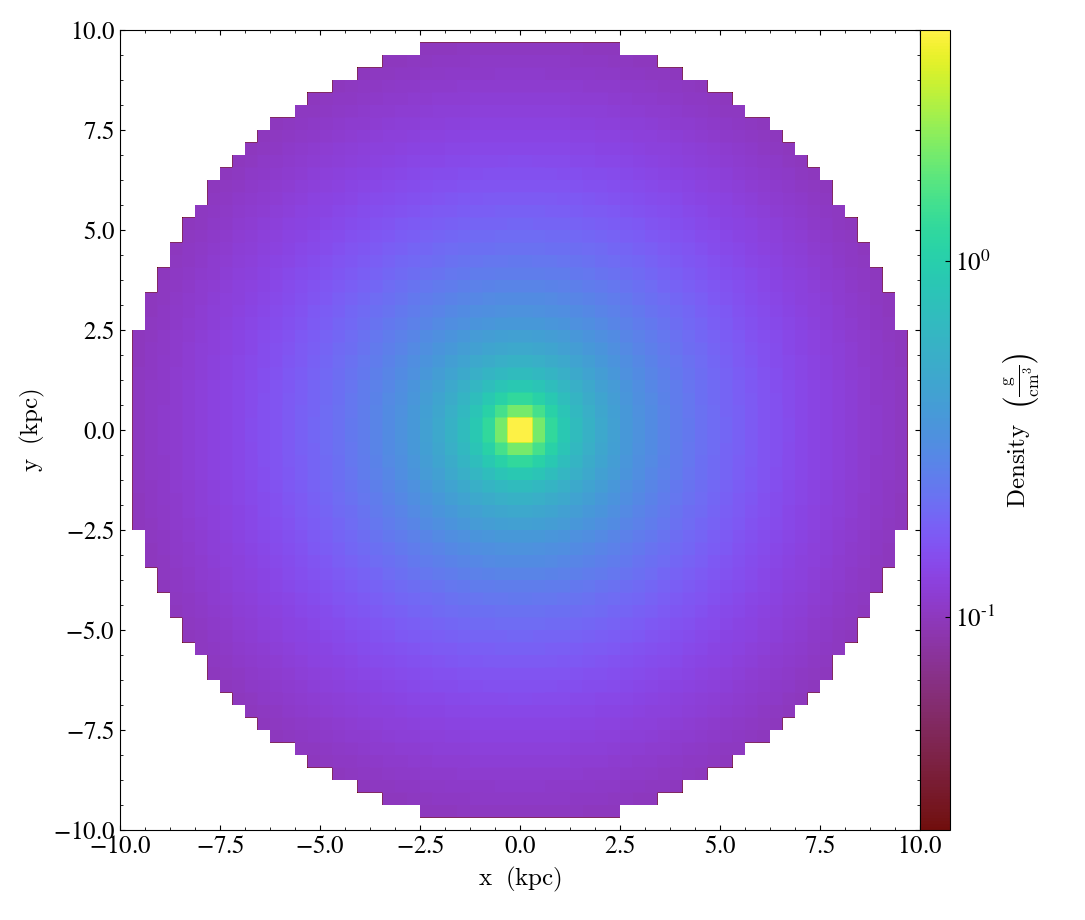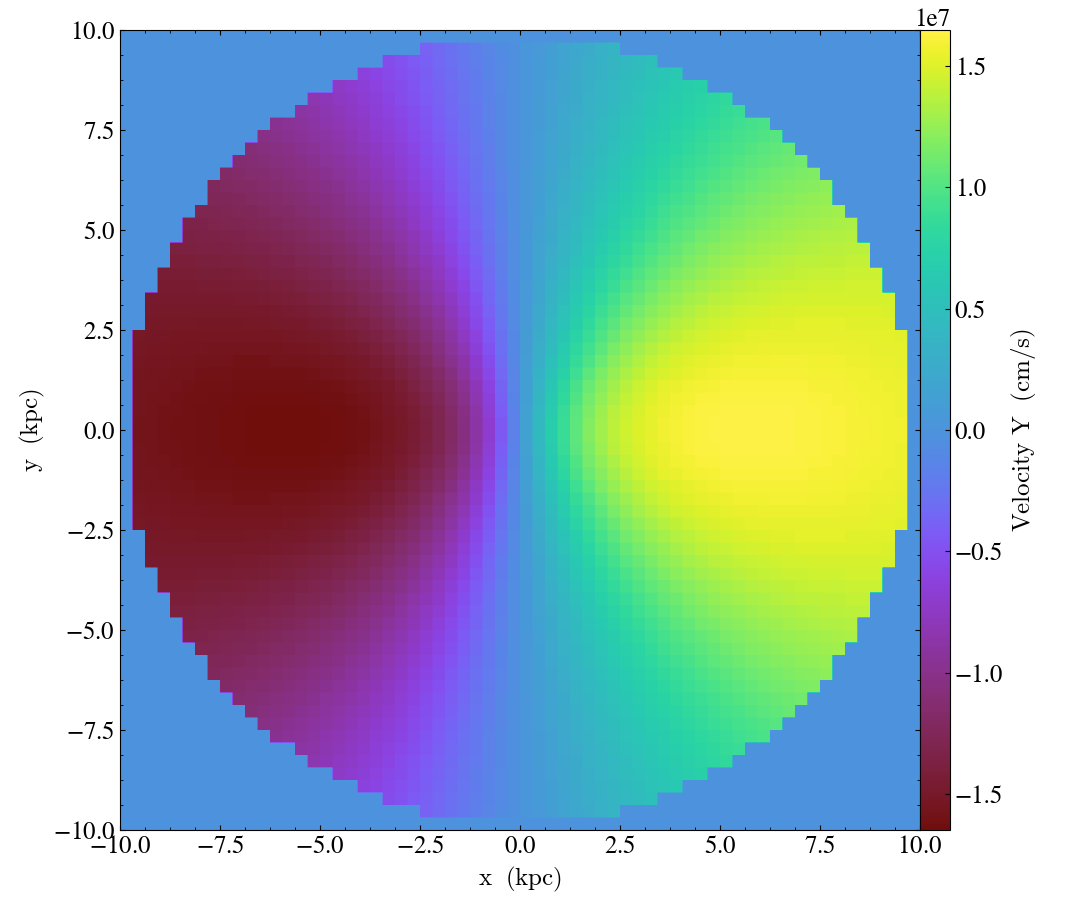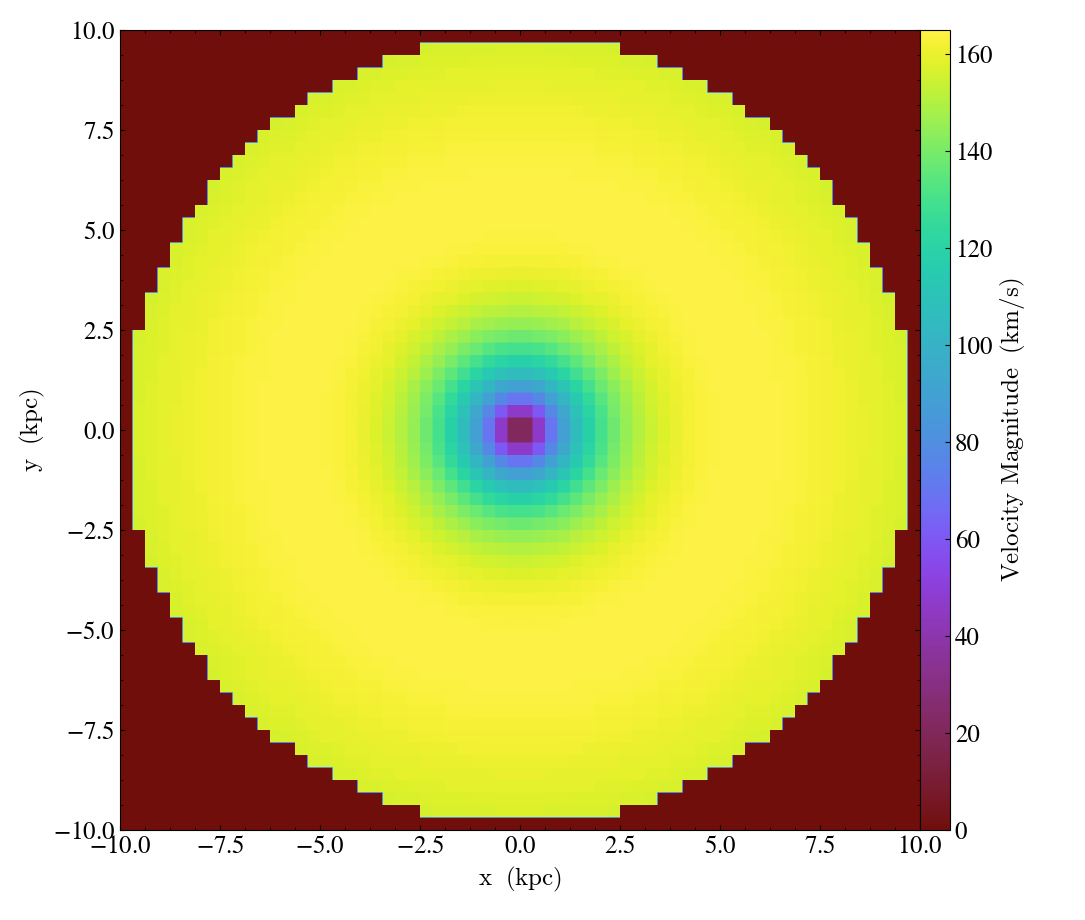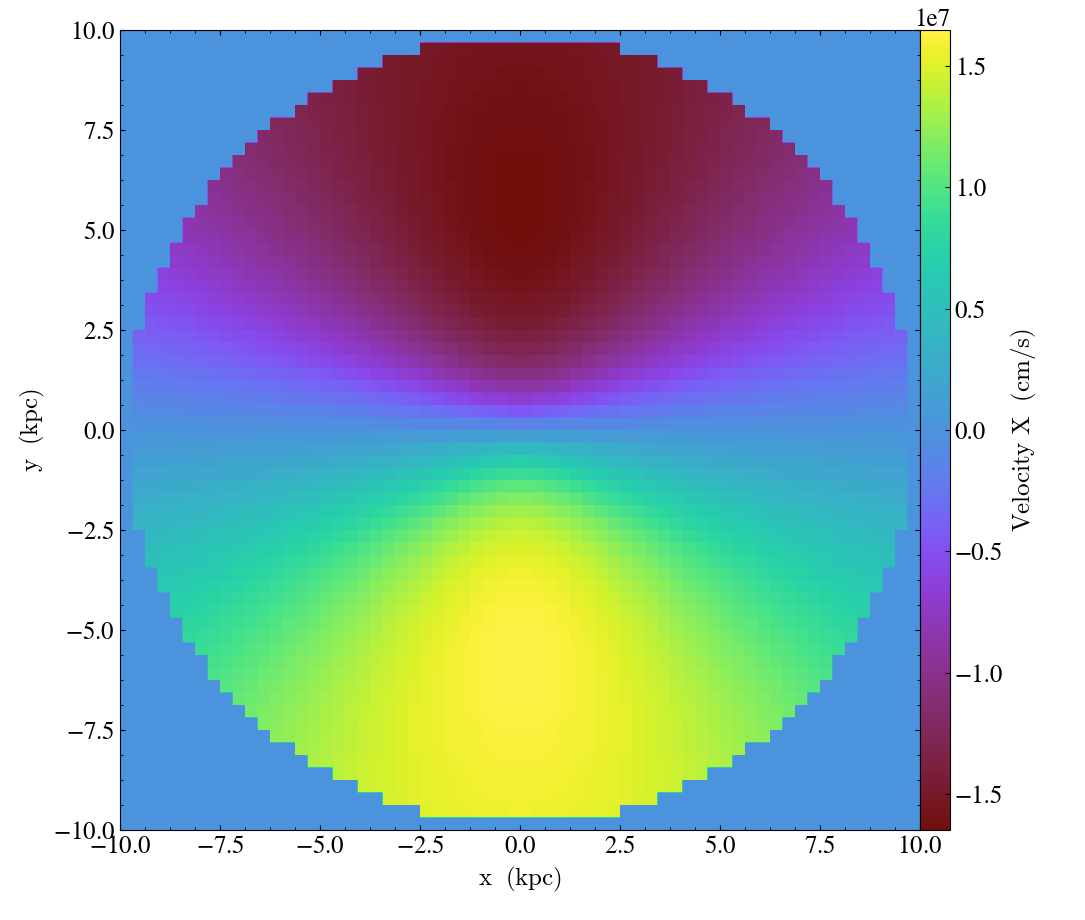Which shows a rotating disk with a specific density and velocity profile. Now, suppose we wanted to look at this disk galaxy from a certain orientation angle, and simulate a 3D FITS data cube where we can see the gas that is emitting at different velocities along the line of sight. We can do this using the PPVCube class. First, let's assume we rotate our viewing angle 60 degrees from face-on, from along the z-axis into the x-axis. We'll create a normal vector:

In :
i = 60.*np.pi/180.
L = [np.sin(i),0.0,np.cos(i)]


Next, we need to specify a field that will serve as the "intensity" of the emission that we see. For simplicity, we'll simply choose the gas density as this field, though it could be any field (including derived fields) in principle. We also need to choose the bounds in line-of-sight velocity that the data will be binned into, which is a 4-tuple in the shape of (vmin, vmax, nbins, units), which specifies a linear range of nbins velocity bins from vmin to vmax in units of units. We may also optionally specify the dimensions of the data cube with the dims argument.

In :
cube = PPVCube(ds, L, "density", (-150.,150.,50,"km/s"), dims=200, method="sum")


Following this, we can now write this cube to a FITS file. The x and y axes of the file can be in length units, which can be optionally specified by length_unit:

In :
cube.write_fits("cube.fits", clobber=True, length_unit="kpc")

/tmp/yt/yt/utilities/parallel_tools/parallel_analysis_interface.py:319: VisibleDeprecationWarning: The "clobber" keyword argument is deprecated. Use the "overwrite" argument, which has the same effect, instead.
return func(*args, **kwargs)


Or one can use the sky_scale and sky_center keywords to set up the coordinates in RA and Dec:

In :
sky_scale = (1.0, "arcsec/kpc")
sky_center = (30., 45.) # RA, Dec in degrees
cube.write_fits("cube_sky.fits", clobber=True, sky_scale=sky_scale, sky_center=sky_center)


Now, we'll look at the FITS dataset in yt and look at different slices along the velocity axis, which is the "z" axis:

In :
ds_cube = yt.load("cube.fits")

In :
# Specifying no center gives us the center slice
slc = yt.SlicePlot(ds_cube, "z", ["density"])
slc.show()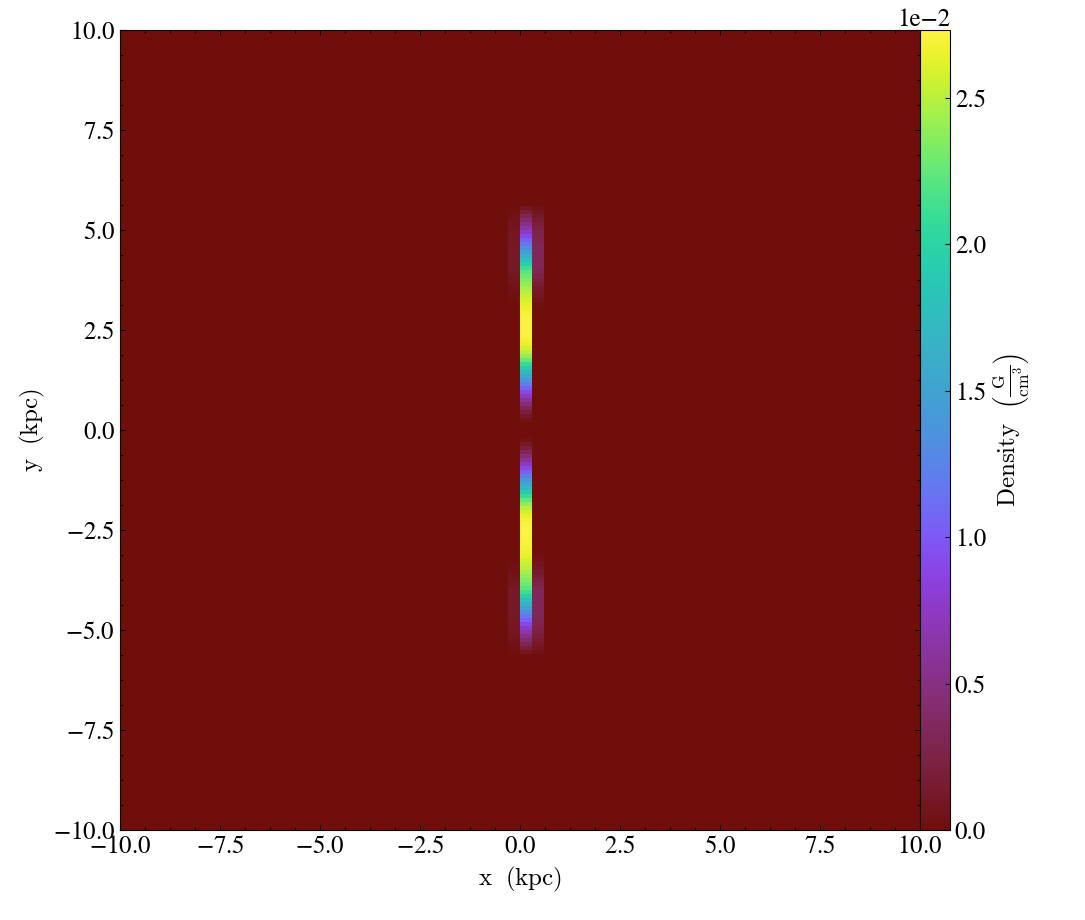In :
# Picking different velocities for the slices
new_center = ds_cube.domain_center
new_center = ds_cube.spec2pixel(-100.*u.km/u.s)
slc = yt.SlicePlot(ds_cube, "z", ["density"], center=new_center)
slc.show()

---------------------------------------------------------------------------
AttributeError                            Traceback (most recent call last)
<ipython-input-13-7097ae0caedd> in <module>
1 # Picking different velocities for the slices
2 new_center = ds_cube.domain_center
----> 3 new_center = ds_cube.spec2pixel(-100.*u.km/u.s)
4 slc = yt.SlicePlot(ds_cube, "z", ["density"], center=new_center)
5 slc.show()

AttributeError: 'FITSDataset' object has no attribute 'spec2pixel'
In :
new_center = ds_cube.spec2pixel(70.0*u.km/u.s)
slc = yt.SlicePlot(ds_cube, "z", ["density"], center=new_center)
slc.show()

---------------------------------------------------------------------------
AttributeError                            Traceback (most recent call last)
<ipython-input-14-2af093200263> in <module>
----> 1 new_center = ds_cube.spec2pixel(70.0*u.km/u.s)
2 slc = yt.SlicePlot(ds_cube, "z", ["density"], center=new_center)
3 slc.show()

AttributeError: 'FITSDataset' object has no attribute 'spec2pixel'
In :
new_center = ds_cube.spec2pixel(-30.0*u.km/u.s)
slc = yt.SlicePlot(ds_cube, "z", ["density"], center=new_center)
slc.show()

---------------------------------------------------------------------------
AttributeError                            Traceback (most recent call last)
<ipython-input-15-5562e2bd6a69> in <module>
----> 1 new_center = ds_cube.spec2pixel(-30.0*u.km/u.s)
2 slc = yt.SlicePlot(ds_cube, "z", ["density"], center=new_center)
3 slc.show()

AttributeError: 'FITSDataset' object has no attribute 'spec2pixel'

If we project all the emission at all the different velocities along the z-axis, we recover the entire disk:

In :
prj = yt.ProjectionPlot(ds_cube, "z", ["density"], method="sum")
prj.set_log("density", True)
prj.set_zlim("density", 1.0e-3, 0.2)
prj.show()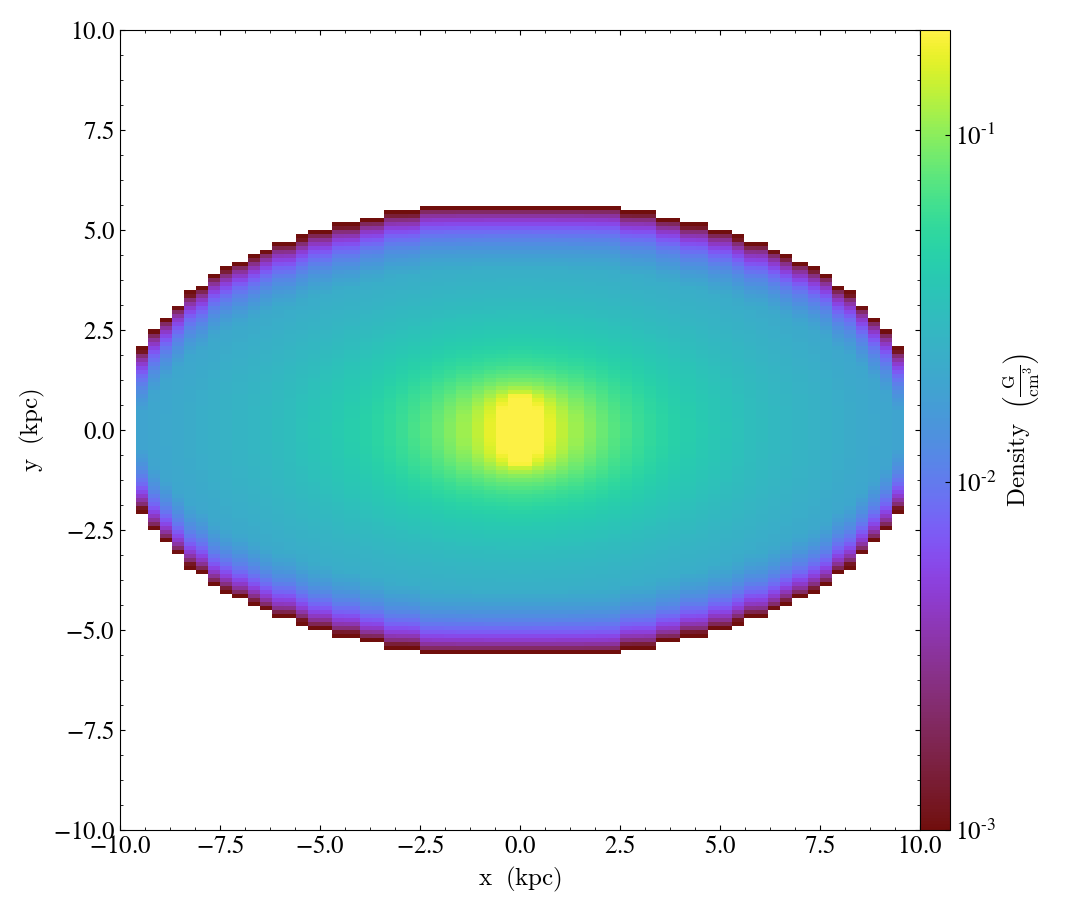The thermal_broad keyword allows one to simulate thermal line broadening based on the temperature, and the atomic_weight argument is used to specify the atomic weight of the particle that is doing the emitting.

In :
cube2 = PPVCube(ds, L, "density", (-150.,150.,50,"km/s"), dims=200, thermal_broad=True,
atomic_weight=12.0, method="sum")
cube2.write_fits("cube2.fits", clobber=True, length_unit="kpc")

/tmp/yt/yt/utilities/parallel_tools/parallel_analysis_interface.py:319: VisibleDeprecationWarning: The "clobber" keyword argument is deprecated. Use the "overwrite" argument, which has the same effect, instead.
return func(*args, **kwargs)


Taking a slice of this cube shows:

In :
ds_cube2 = yt.load("cube2.fits")
new_center = ds_cube2.domain_center
new_center = ds_cube2.spec2pixel(70.0*u.km/u.s)
slc = yt.SlicePlot(ds_cube2, "z", ["density"], center=new_center)
slc.show()

---------------------------------------------------------------------------
AttributeError                            Traceback (most recent call last)
<ipython-input-18-4ac13a4044cd> in <module>
2 new_center = ds_cube2.domain_center
----> 3 new_center = ds_cube2.spec2pixel(70.0*u.km/u.s)
4 slc = yt.SlicePlot(ds_cube2, "z", ["density"], center=new_center)
5 slc.show()

AttributeError: 'FITSDataset' object has no attribute 'spec2pixel'
In :
new_center = ds_cube2.spec2pixel(-100.*u.km/u.s)
slc = yt.SlicePlot(ds_cube2, "z", ["density"], center=new_center)
slc.show()

---------------------------------------------------------------------------
AttributeError                            Traceback (most recent call last)
<ipython-input-19-1a3178f6761b> in <module>
----> 1 new_center = ds_cube2.spec2pixel(-100.*u.km/u.s)
2 slc = yt.SlicePlot(ds_cube2, "z", ["density"], center=new_center)
3 slc.show()

AttributeError: 'FITSDataset' object has no attribute 'spec2pixel'

where we can see the emission has been smeared into this velocity slice from neighboring slices due to the thermal broadening.

Finally, the "velocity" or "spectral" axis of the cube can be changed to a different unit, such as wavelength, frequency, or energy:

In :
print (cube2.vbins, cube2.vbins[-1])
cube2.transform_spectral_axis(400.0,"nm")
print (cube2.vbins, cube2.vbins[-1])

-150.0 km/s 150.0 km/s
399.79996163130727 nm 400.20023864575296 nm


If a FITS file is now written from the cube, the spectral axis will be in the new units. To reset the spectral axis back to the original velocity units:

In :
cube2.reset_spectral_axis()
print (cube2.vbins, cube2.vbins[-1])

-150.0 km/s 150.0 km/s